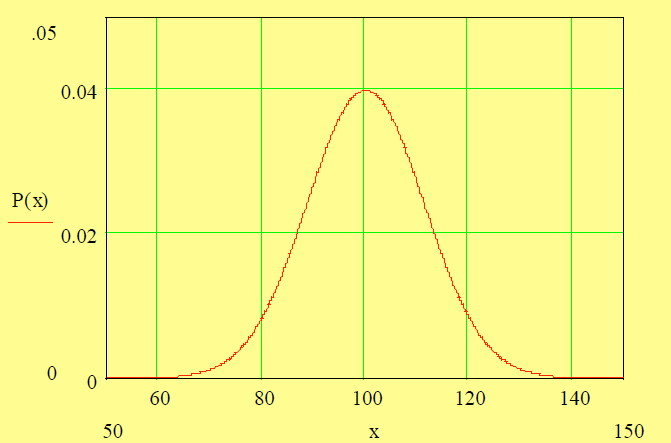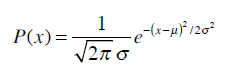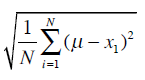Special Internshala Offer! Get Flat 80% Off on all Internshala Training Programs! All Courses @ Rs.999 Enroll Now!

# Errors in Measurements | Introduction to Surveying | Explanatory Video | By Ashutosh Nautiyal

Errors in Measurement In practice, we can never measure the true value of any length, angle or any geoinformation because there will always be some error in the measurement. Sources of Error in Measurement Natural Sources (like temperature, wind, etc.) The instrument being used (there may be some error in the measurement of the instrument) The person taking the measurement (the person may introduce some error in the measurement)

## Errors in Measurement

In practice, we can never measure the true value of any length, angle or any geoinformation because there will always be some error in the measurement.

## Sources of Error in Measurement:

• Natural Sources (like temperature, wind, etc.)

• The instrument being used (there may be some error in the measurement of the instrument)

• The person taking the measurement (the person may introduce some error in the measurement)

## Blunder or Mistake

• It occurs because of carelessness.

## Systematic Error

• It is caused due to systematic forces.

• These errors follow some physical law (as in the case of temperature, gravity, etc.).

• We can write the mathematical model for these errors.

• These errors can be eliminated.

## Random Error

• These errors are caused by many sources which we cannot account for.

• They are equally distributed in both the positive and negative direction (or their probability of occurrence in both the positive and negative direction is the same).

• Random errors which are larger in magnitude occur less while random errors which are smaller in magnitude occur more.

• After knowing that the observation is not a blunder or mistake and eliminating systematic errors from it, there are still random errors in it.

## Precision

• The meaning of Precision is the closeness of observations.

• If the systematic errors are eliminated, the precision of the observations become an indicator of the accuracy.

## Accuracy

• The meaning of Accuracy is the closeness of our observation to the true value.

## True Error

• True error is defined as the difference between the true value and the observed value.

• True error = True value (L)- Observed Value (l)

## Relative Error

• In one of the cases, for a scale, the relative error is defined as the ratio of the error which is being introduced because of the least count to the observed value.

• Relative error = [that error (Δl) / observed value (l)]

## Percentage Error

• The relative error multiplied by 100 gives the value of the percentage error.

• Percentage error = Relative error * 100

## Most Probable Value (MPV)

• It is defined as the value which has the maximum probability of being nearest to the true value.

• It is a kind of replacement for the true value.

• MPV = Arithmetic Mean

• x̄ = [(x1 + x2 + x3 + .................. + xn) / n)] = MPV

## Residual

• For any observation x,

• Residual (r) = MPV (x̄) - Any observed value (x)

## Proof for MPV= Arithmetic Mean

Since, r = x̄ - x

So, r1 =  x̄ - x1

Similarly, r2 =  x̄ - x2 , r3 =  x̄ - x3 .....and so on.

and rn =  x̄ - xn

On adding the values of r (from r1 to rn), we get;

r1 + r2 + r3 + r4 + ......... + rn =  (x̄*n) - (x1 + x2 + x3 + ............. + xn)

Now, in the case when all the observations have only random errors;

For large n, (r1 + r2 + r3 + ...... + rn) = 0 (because the random errors have got equal probability of going into the positive as well as in the negative direction).

Now, (x̄*n) = x1 + x2 + x3 + ............. + xn

Therefore, MPV (x̄) = [(x1 + x2 + x3 + ............. + xn) / n)] = Arithmetic Mean

Hence Proved.

## Random Error Distribution

• The random error distribution curve is called the Normal curve or Gaussian curve or bell-shaped curve.

• There is an equal probability of observation or error to go in both the positive and negative direction of the Most Probable Value.

• Our observations or our errors are equally distributed in both the positive and negative side.

• The errors which are large in magnitude have a smaller probability of occurrence and the errors which are small in magnitude have a larger probability of occurrence.

• The probability of occurrence of the Maximum Probable Value (MPV) is maximum.Gaussian Curve or Normal Curve

The Probability density function for the Gaussian Curve is given by:-Probability density function P(x)

where μ is MPV

x is an observation and a variable

P(x) is the probability of occurrence of the variable x or Probability density function

σ is Standard deviation which is =*Self Typed
*Source - Internet, Books, Self-Analysis

Getting Info...